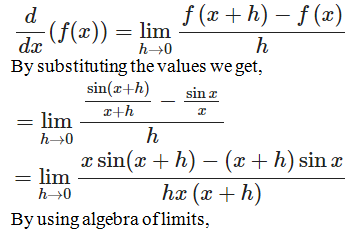• +91 9971497814
• info@interviewmaterial.com

# RD Chapter 30- Derivatives Ex-30.2 Interview Questions Answers

### Related Subjects

Question 1 :

Differentiate eachof the following from first principles:
(i) 2/x
(ii) 1/√x

(iii) 1/x3

(iv) [x2 +1]/ x

(v) [x2 –1] / x

(i) 2/x
Given:
f (x) = 2/x
By using the formula,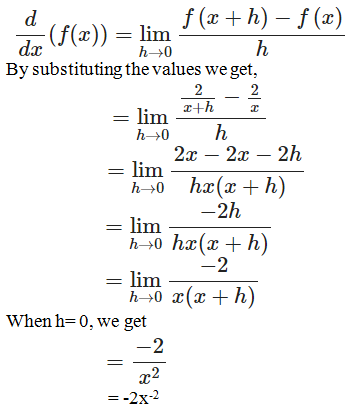∴ Derivative of f(x) = 2/x is -2x-2
(ii) 1/√x
Given:
f (x) = 1/√x
By using the formula,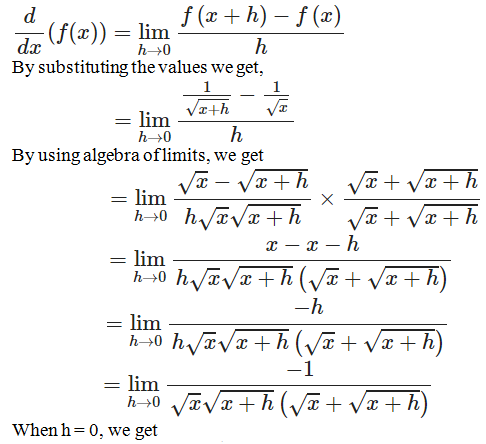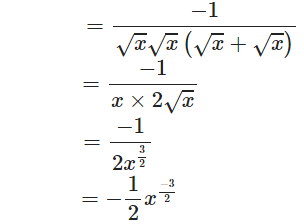Derivativeof f(x) = 1/√x is -1/2 x-3/2

(iii) 1/x3

Given:

f (x) = 1/x3

By using the formula,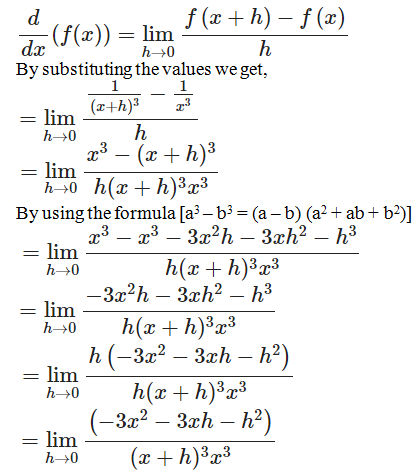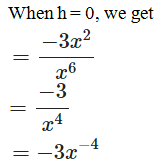Derivative of f(x) = 1/x3 is -3x-4

(iv) [x2 + 1]/ x

Given:

f (x) = [x2 + 1]/x

By using the formula,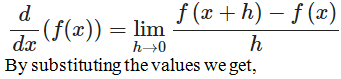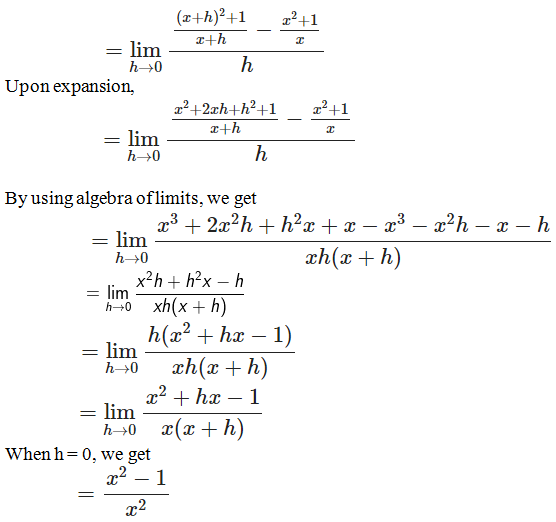= 1 – 1/x2

Derivative of f(x) = 1 – 1/x2

(v) [x2 – 1] / x

Given:

f (x) = [x2 – 1]/x

By using the formula,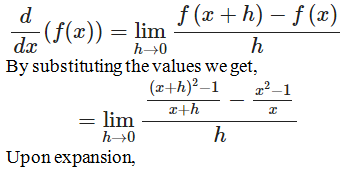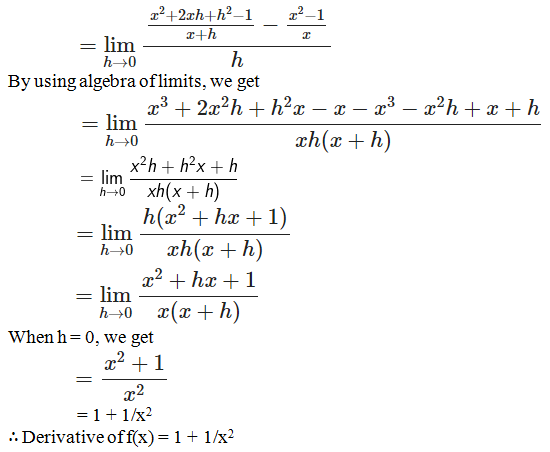Question 2 :

Differentiate eachof the following from first principles:

(i) e-x

(ii) e3x

(iii) eax+b

(i) e-x

Given:

f (x) = e-x

By using the formula,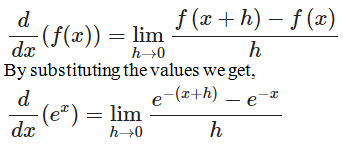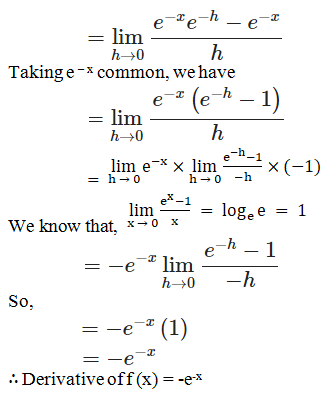(ii) e3x

Given:

f (x) = e3x

By using the formula,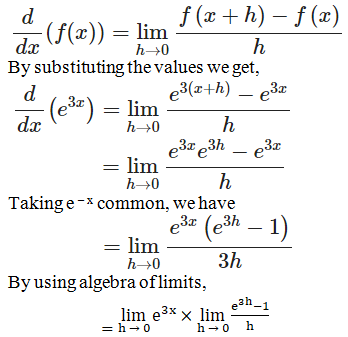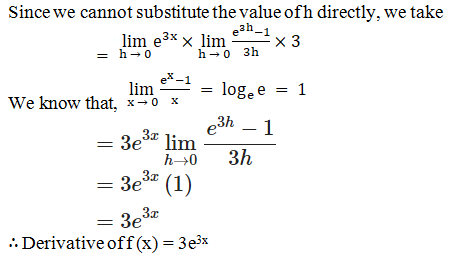(iii) eax+b

Given:

f (x) = eax+b

By using the formula,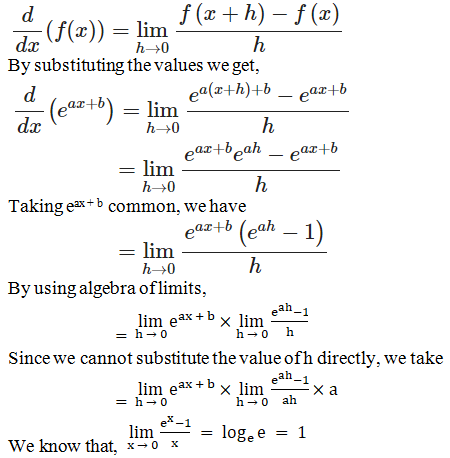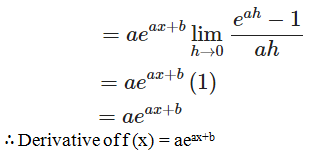Question 3 :
Differentiate each of the following from first principles:
(i) √(sin 2x)
(ii) sin x/x

(i) √(sin 2x)
Given:
f (x) = √(sin 2x)
By using the formula,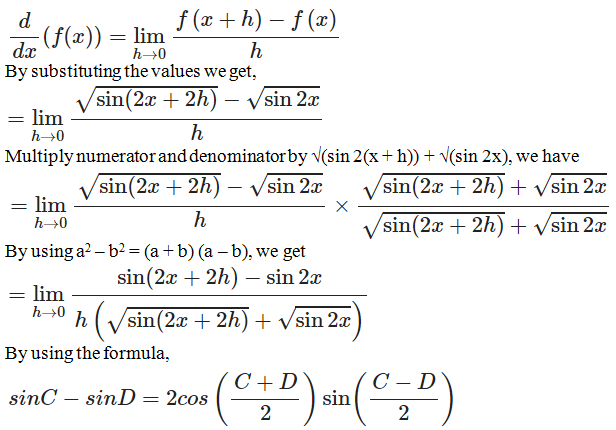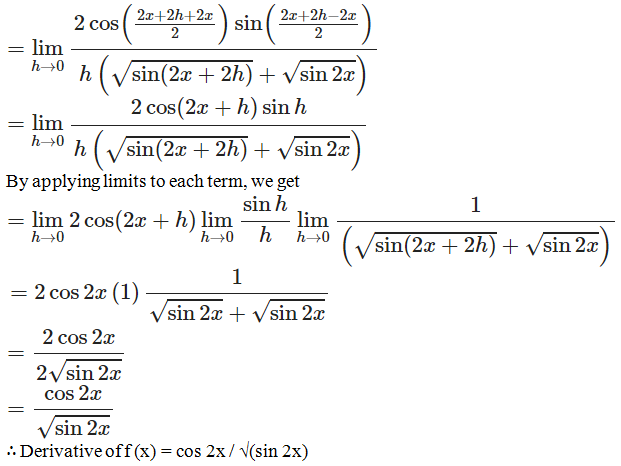(ii) sin x/x

Given:

f (x) = sin x/x

By using the formula,